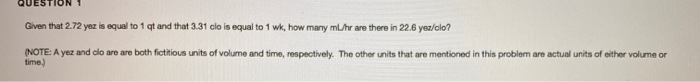# Question Solved1 AnswerQUESTION 1 Given that 2.72 yez is equal to 1 qt and that 3.31 clo is equal to 1 wk, how many ml/hr are there in 22.6 yea/clo? (NOTE: A yez and clo are are both fictitious units of volume and time, respectively. The other units that are mentioned in this problem are actual units of either volume or time)Transcribed Image Text: QUESTION 1 Given that 2.72 yez is equal to 1 qt and that 3.31 clo is equal to 1 wk, how many ml/hr are there in 22.6 yea/clo? (NOTE: A yez and clo are are both fictitious units of volume and time, respectively. The other units that are mentioned in this problem are actual units of either volume or time)
More
Transcribed Image Text: QUESTION 1 Given that 2.72 yez is equal to 1 qt and that 3.31 clo is equal to 1 wk, how many ml/hr are there in 22.6 yea/clo? (NOTE: A yez and clo are are both fictitious units of volume and time, respectively. The other units that are mentioned in this problem are actual units of either volume or time)